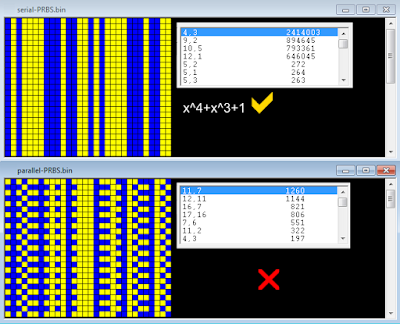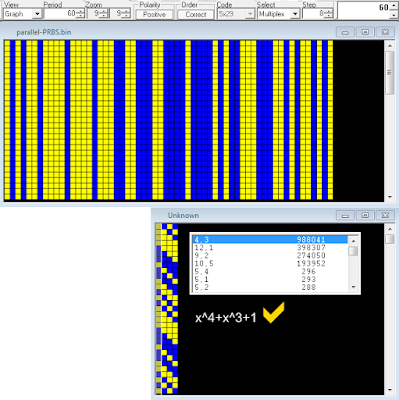## 6 August 2021

### another approach to the analysis of unresolved sync sequences

The pseudo-random binary sequences (PRBS, also referred to as pseudo-random bit sequences) are largely used in telecommunication (data scrambling, sync sequences, field delimiter markers, jamming, stream ciphers, bit-error rate tests, ...) and are usually generated by physical Linear Feedback Shift Registers (LFSRs), or their software implementations. Sometimes it may happen to come across sync sequences or particular patterns of which it is not possible to trace back to the generator polynomial and therefore to the structure of the used LFSR. This happens because most of the time PRBS are drained from the serial output of the LFSR and therefore the search for the generator polynomial takes place brute-force and "directly" on the pattern being analyzed.Fig. 1 - x^4+x^3+1 LFSR with serial output

But it could happen that the pseudo-random sequence is instead formed by taking the outputs (the states) of all the stages that compose the LFSR, let's say a parallel-output PRBS as an alternative counterpart to the serial-output one.Fig. 2 - x^4+x^3+1 LFSR with parallel outputs

In the first case (serial-output PRBS) the sequence has a length (or period T) of 15 bit while in the second case (parallel-output PRBS) the sequence has a period  of 15×4=60 bit length. Analyzing the latter as if it were a serial-output one could produce not significant or even misleading results, as shown in figure 3.Fig. 3 - results after analyzing the serial and parallel output of the same LFSR (x^4+x^3+1)

What we need is establish the number of stages that compose the unknown LFSR, after which a single stage can be isolated and its (serial) output sequence analyzed. Well, assuming T is the period of the pattern, this one shall be reshaped to a c-columns array where c = T/((2^n)-1), where n = (2,3,4,5,6,7...).  After reshaped the original pattern, the search for the generator polynomial can be performed on any of the c columns of that array.  In a few words, we have to found the valor the period T in table below (if any) and then reshape the pattern according to the corrispondent value of c (ie, the number of the stages of the LFSR):

In case of the x^4+x^3+1 LFSR mentioned above, its parallel output has a period length of 60 bit and  is divisible by 15, giving 4 as the number of the stages: the polynomial is then easily verified after reshaping the 60-bit pattern to a 4-bit array (figure 4). Notice that the the length of the serial-output PRBS is just equal to 15.Fig. 4

Other examples are shown below:

Obviously, the above is valid only for certain lengths of the period T and it is not certain that it is also valid for generator polynomials that are not primitive, i.e. they do not generate sequences of maximum length . Moreover, it could be argued that a multiplexer is needed to send the parallel-output PRBS, but the whole sequence could also be stored and then pre-loaded and added to the bitstream as many times as needed, or it could happen that only a segment of a parallel-output PRBS could be used.

I am definitely not a "mathematician" but I think that sometimes the above method, though a bit raw, can be useful to unearth the LFSR which is hidden behind particular sequences.